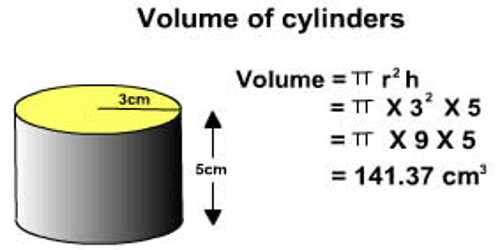Volume of Cylinder

A cylinder is a solid composed of two congruent circles in parallel planes, their interiors, and all the line segments parallel to the segment containing the centers of both circles with endpoints on the circular regions.

The volume of a cylinder equals the (area of the base)*height = π r2 h

where:

• π is Pi, approximately 3.142
• r is the radius of the circular end of the cylinder
• h height of the cylinder

The volume of a 3 -dimensional solid is the amount of space it occupies. Volume is measured in cubic units (in3, ft3, cm3, m3 etc). Be sure that all of the measurements are in the same unit before computing the volume.Example:

Find the volume of the cylinder shown. Round to the neatest cubic centimeter.

The formula for the volume of a cylinder is V = Bh  or  V = πr2 h  or  V= πr2 h .

The radius of the cylinder is 88 cm and the height is 15 cm.

Substitute 88 for rr and 1515 for hh in the formula V=πr2h

V= π(8)2 (15)

Simplify.

V = π(64)(15) ≈ 3016

Therefore, the volume of the cylinder is about 3016 cubic centimeters.

Information Source: